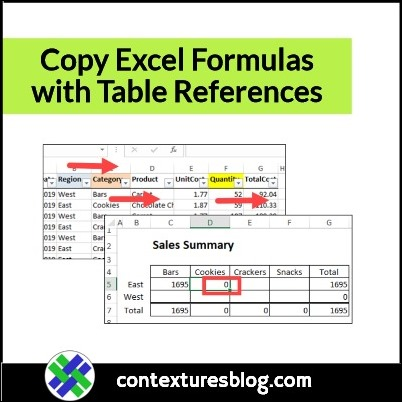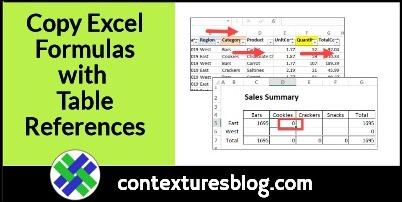## How to Copy Excel Formulas with Table References

Written by Debra Dalgleish from Contextures Blog

If you create Excel formulas that include table references, and then try to copy those formulas to adjacent columns, you might get incorrect results. See why that happens, and how to avoid those problems, when you copy Excel formulas with table references.

### Problem Copying Formulas with Table References

This video shows the problem when copying formulas with table references, and two ways to prevent it. There are written steps below the video.

Video Timeline

• 00:00 Introduction
• 00:46 SUBTOTAL Formula to Check Totals
• 01:19 SUMIFS Formula with Table References
• 01:42 Copy the Formula Down One Row
• 02:13 Copy Across
• 03:09 Copy With No Problems

### Excel Formula with Table References

In the video, there’s a sales summary, with a SUMIFS formula in cell C5. That formula shows the correct total for Bars sales in the East region.Here’s the formula in cell C5:

=SUMIFS(Sales_Data[Quantity], Sales_Data[Region],\$B5, Sales_Data[Category],C\$4)

The formula refers to heading cells in the sales summary:

• Region name – \$B5
• Category name – C\$4

The formula also refers to 3 columns in the Sales_Data table, which is on a different worksheet:

• Sales_Data[Quantity]
• Sales_Data[Region]
• Sales_Data[Category]

### Copy the Formula to Next Column

The formula in cell C5 is working correctly, but see what happens if you try these steps:

• Select cell C5, and point to the fill handle, in the cells bottom right corner
• When the pointer changes to a black plus sign, drag right, to put the formula in cell D5 (East – Cookies)

The formula in cell D5 shows an incorrect total of zero. If you check the sales data, the quantity for East Cookie sales is  1425.### Table References Shifted Right

To troubleshoot the problem, click cell D5, at look in the formula bar.

Instead of showing the same formula that was in cell C5, the table references have changed.

=SUMIFS(Sales_Data[TotalCost], Sales_Data[Category],\$B5, Sales_Data[Product],D\$4)

• Quantity changed to Total Cost
• Region changed to Category
• Category changed to ProductEast isn’t found in the Category column and Cookies isn’t found in the Product column, so the SUMIFS formula result is zero.

### Prevent Problems with Table References

To prevent this problem of shifting table references, don’t use the fill handle to copy a formula across columns.

Instead, use one of the following methods – Fill Right, or Copy and Paste. The steps are shown below.:

#### Fill Right

• Select the cell with the formula, and the cells to the right, where you want to copy the formula – cells C5:F5 in the screen shot below
• Press Ctrl+R to fill the formula to the right#### Copy and Paste

• Select the cell with the formula, and press Ctrl+C to copy it
• Select all the cells where you want to copy the formula
• Press Ctrl+V to paste the formula### Get the Sample File

To get the sample file used in the video, go to the Excel Sum Function Examples page on my Contextures website.

In the Download section, look for the Table References workbook. The zipped file is in xlsx format, and does not contain any macros.

________________

### Copy Excel Formulas with Table References________________

How to Copy Excel Formulas with Table References is a post from Contextures Blog and is not allowed to be copied to other sites